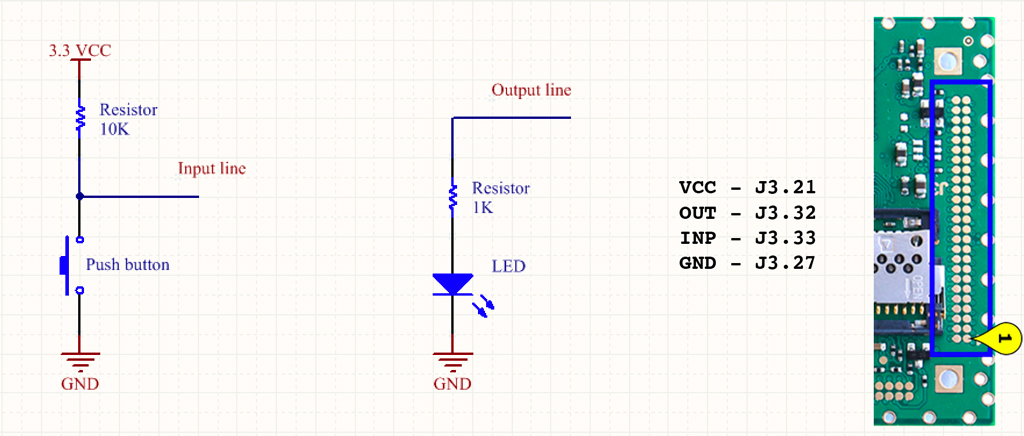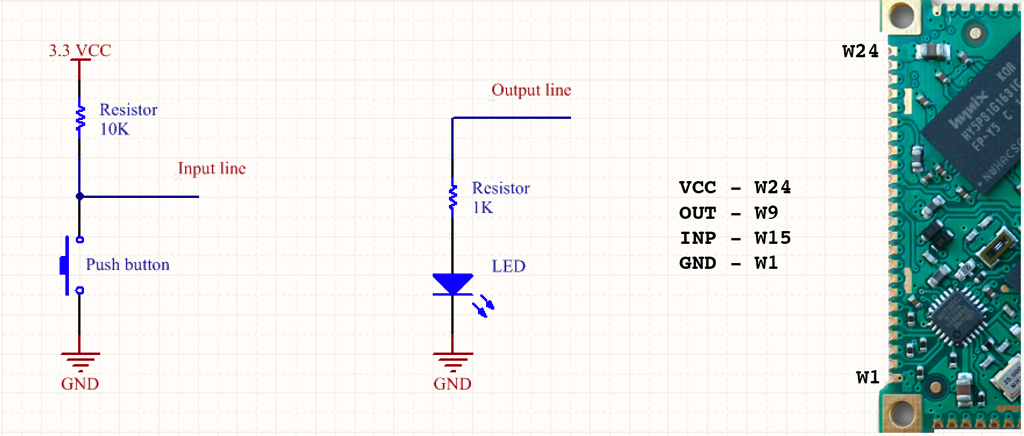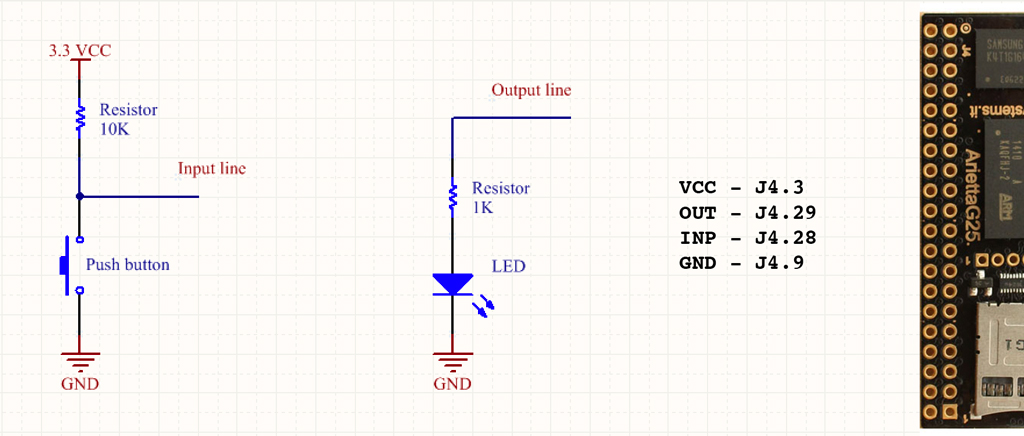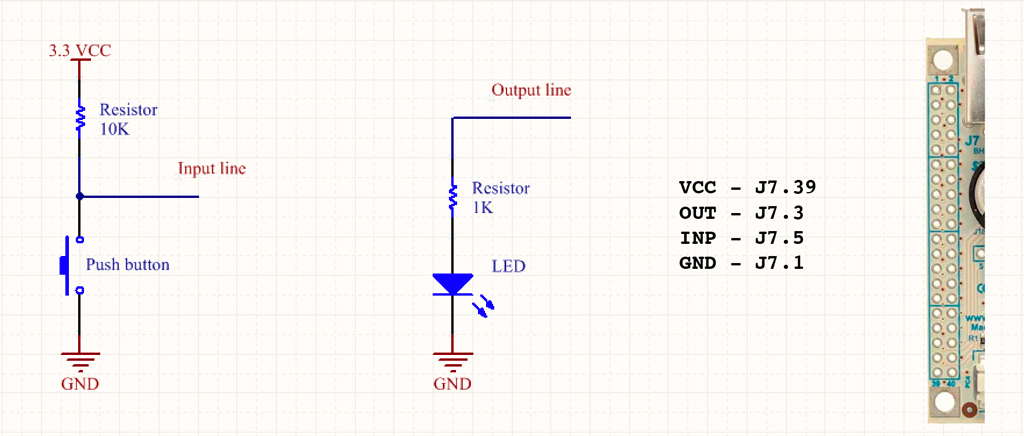## Python examples

A simple module called acmepins is available on GitHub to manage the GPIO lines using the sysfs method.

As shown in the following examples with this module is possible to manage the GPIO states using directly the board pin names or the Atmel chip pin names or the GPIO Kernel IDs.### An output example: a Blinking led

This example will blink a led wired to a output line

```from acmepins import GPIO
from time import sleep

#FOX Board G20 example
led = GPIO('J7.3','OUTPUT')

#Aria G25 example
#led = GPIO('W9','OUTPUT')

#Arietta G25 example
#led = GPIO('J4.29','OUTPUT')

#Acqua A5 example
#led = GPIO('J3.32','OUTPUT')

while True:
led.on()
sleep(1)
led.off()
sleep(1)
```

### An input example: Reading a push button

there are two ways o read a GPIO input state:

• Checking inside a loop the input state (polling mode)
• Setting a callback function for any input state change (event mode)

### Polling mode

```from acmepins import GPIO
from time import sleep

#FOX Board G20 example
Button=GPIO('J7.5','INPUT')

#Aria G25 example
#Button=GPIO('W15','INPUT')

#Arietta G25 example
#Button=GPIO('J4.28','INPUT')

#Acqua A5 example
#Button=GPIO('J3.33','INPUT')

i=0
while True:
sleep(1)
i+=1
print i
if Button.digitalRead()==0:
print "Pressed"
while Button.digitalRead()==0:
pass
```

### Event mode

```from acmepins import GPIO
from time import sleep

def event_handler():
print "Input changed"

#FOX Board G20 example
Button=GPIO('J7.5','INPUT')

#Aria G25 example
#Button=GPIO('W15','INPUT')

#Arietta G25 example
#Button=GPIO('J4.28','INPUT')

#Acqua A5 example
#Button=GPIO('J3.33','INPUT')

Button.set_edge("both",event_handler)

i=0
while True:
print i
i=i+1
sleep(0.5)
```

One of three conditions can be checked:

• falling: the callback function is called when the signal goes from 1 to 0 (Not available on FOX G20)
• rising: the callback function is called when the signal goes from 0 to 1 (Not available on FOX G20)
• both: the callback function is called on any status change